# Exchange Rates and the Foreign Exchange Market Roberto

• Slides: 27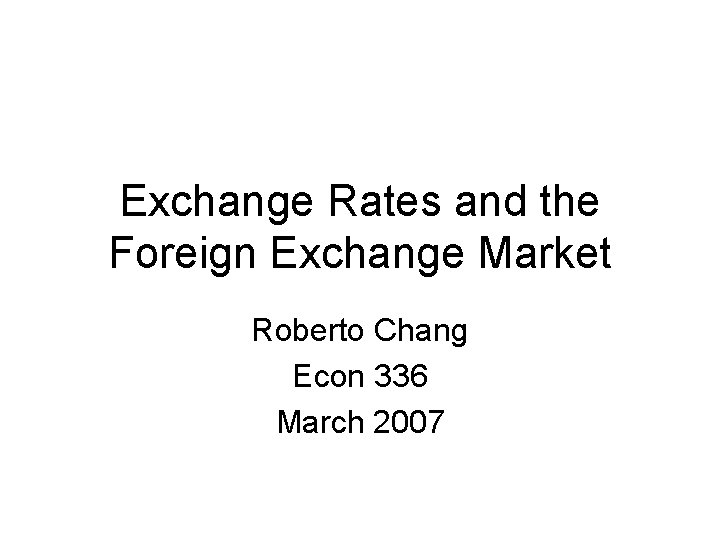Exchange Rates and the Foreign Exchange Market Roberto Chang Econ 336 March 2007Plan • Main reference: chapter 13 of KO • Exchange Rates: definitions, appreciation and depreciation. • The Foreign Exchange Market: actors, contracts. • The concept of rate of return on domestic and foreign currency assets. • Interest rate parity: a first approach to exchange rate determination.Exchange Rates: definition • The exchange rate between two currencies is the price of one currency in terms of the other. • Example: 1 Euro = 1. 30 US dollars The exchange rate is 1. 30 dollars per Euro, or 1/1. 30 Euros per dollar.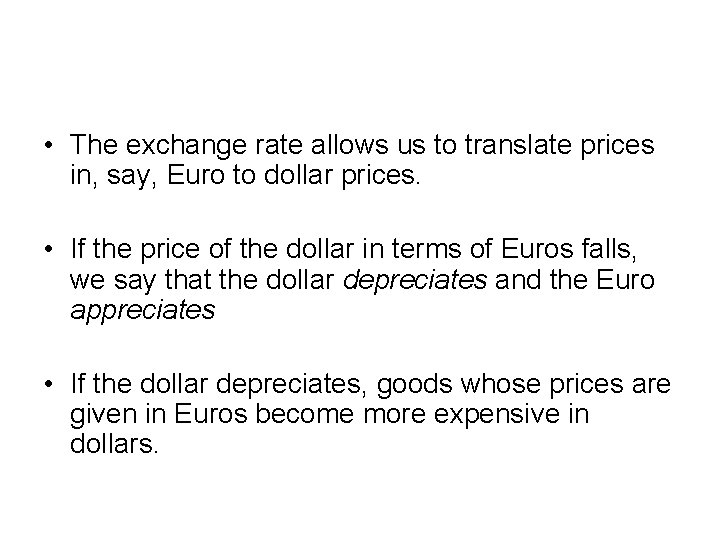• The exchange rate allows us to translate prices in, say, Euro to dollar prices. • If the price of the dollar in terms of Euros falls, we say that the dollar depreciates and the Euro appreciates • If the dollar depreciates, goods whose prices are given in Euros become more expensive in dollars.The Foreign Exchange Market: the Actors • Commercial Banks • Non Bank Financial Institutions • Firms • Central Banks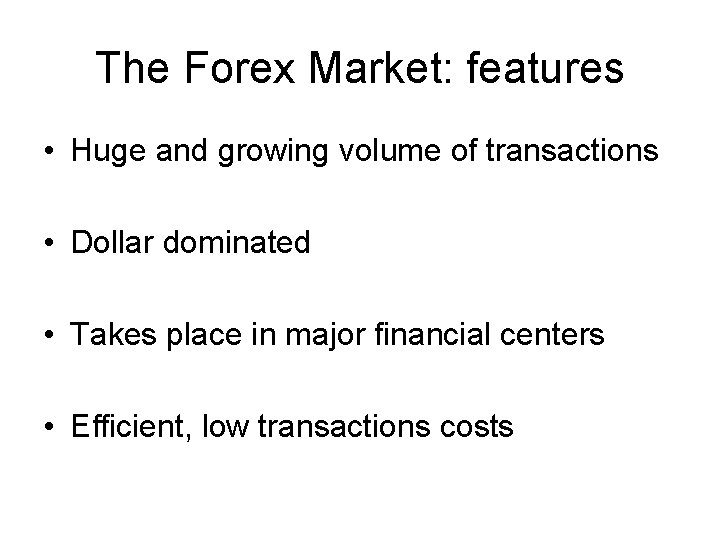The Forex Market: features • Huge and growing volume of transactions • Dollar dominated • Takes place in major financial centers • Efficient, low transactions costsTypes of transactions • Spot • Forward and futures contracts • Swaps • Options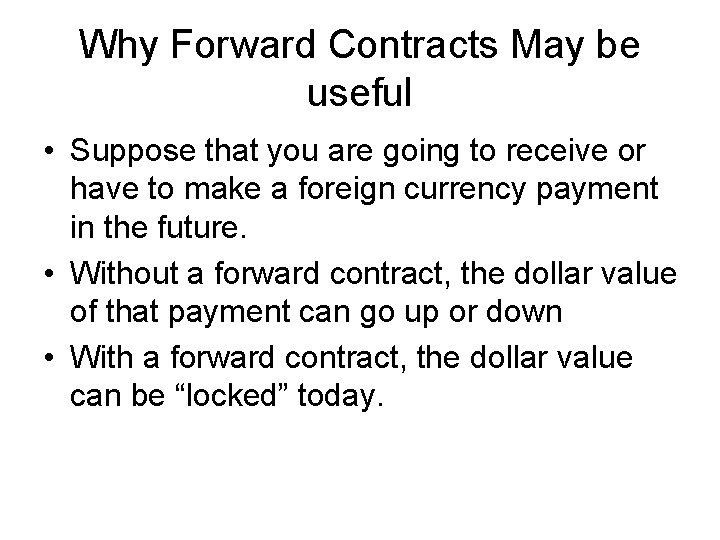Why Forward Contracts May be useful • Suppose that you are going to receive or have to make a foreign currency payment in the future. • Without a forward contract, the dollar value of that payment can go up or down • With a forward contract, the dollar value can be “locked” today.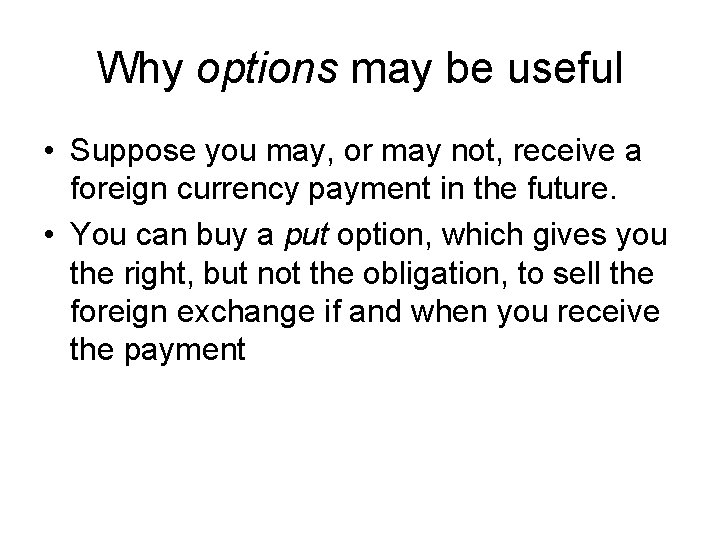Why options may be useful • Suppose you may, or may not, receive a foreign currency payment in the future. • You can buy a put option, which gives you the right, but not the obligation, to sell the foreign exchange if and when you receive the payment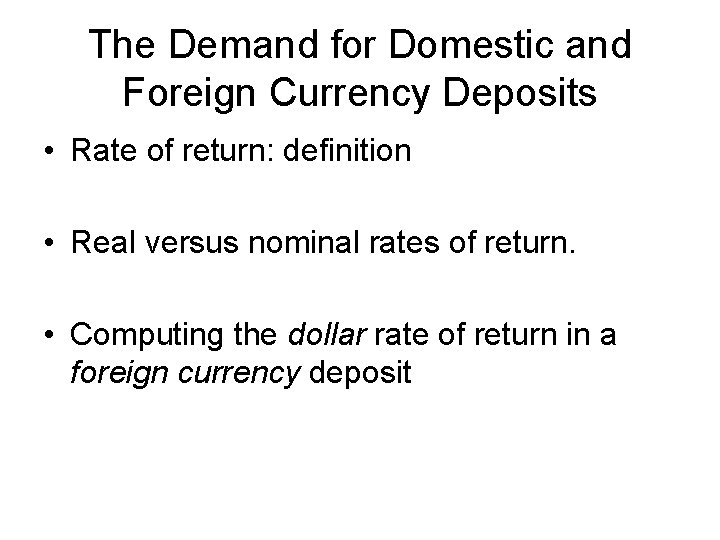The Demand for Domestic and Foreign Currency Deposits • Rate of return: definition • Real versus nominal rates of return. • Computing the dollar rate of return in a foreign currency deposit• The dollar rate of return on a Euro deposit is, approximately, the interest rate on Euro deposits plus the expected rate of depreciation of the dollar against the EuroInterest parity • We will assume that: i = i* + (e’-e)/e where i = interest rate on domestic currency deposits i* = interest rate on foreign currency deposits e = current exchange rate e’ = future expected exchange rate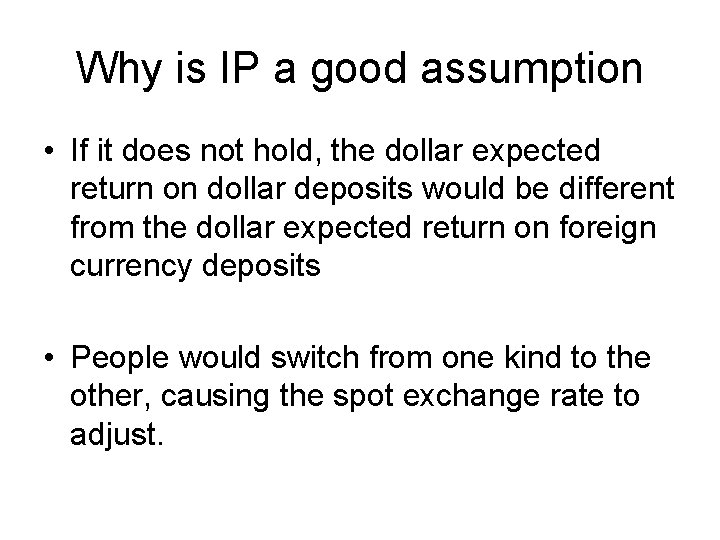Why is IP a good assumption • If it does not hold, the dollar expected return on dollar deposits would be different from the dollar expected return on foreign currency deposits • People would switch from one kind to the other, causing the spot exchange rate to adjust.Expected Returns on Euro Deposits when Ee\$/€ = \$1. 05 Per Euro Expected rate of Current Interest rate on dollar exchange rate euro deposits depreciation E\$/€ R€ (1. 05 - E\$/€)/E\$/€ Expected dollar return on euro deposits R€ + (1. 05 - E\$/€)/E\$/€ 1. 07 0. 05 -0. 019 0. 031 1. 05 0. 000 0. 050 1. 03 0. 05 0. 019 0. 069 1. 02 0. 05 0. 029 0. 079 1. 00 0. 050 0. 100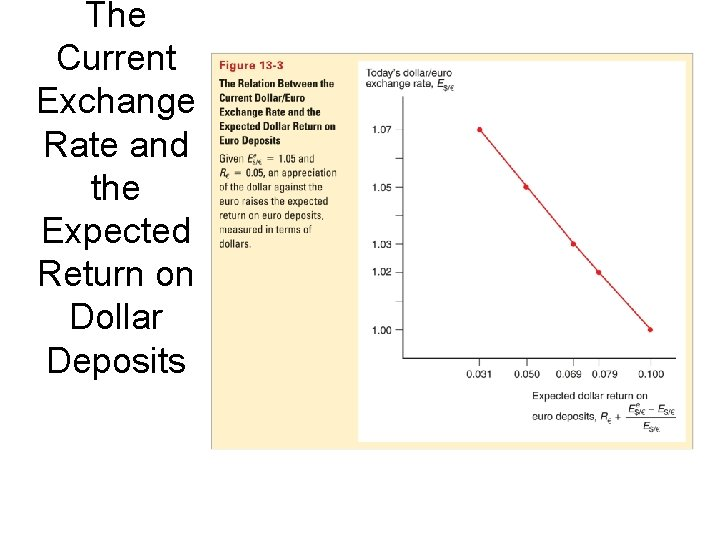The Current Exchange Rate and the Expected Return on Dollar Deposits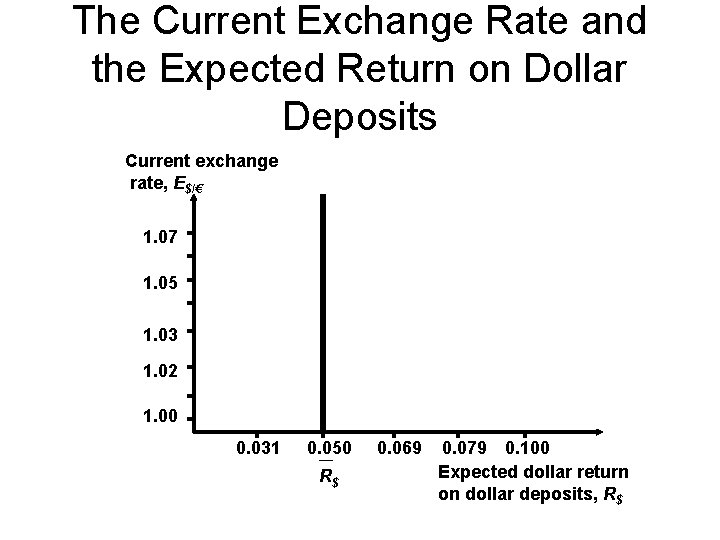The Current Exchange Rate and the Expected Return on Dollar Deposits Current exchange rate, E\$/€ 1. 07 1. 05 1. 03 1. 02 1. 00 0. 031 0. 050 R\$ 0. 069 0. 079 0. 100 Expected dollar return on dollar deposits, R\$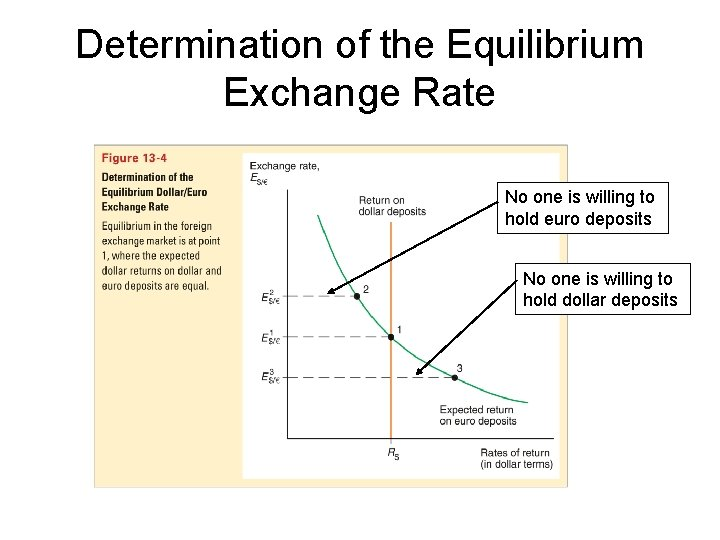Determination of the Equilibrium Exchange Rate No one is willing to hold euro deposits No one is willing to hold dollar deposits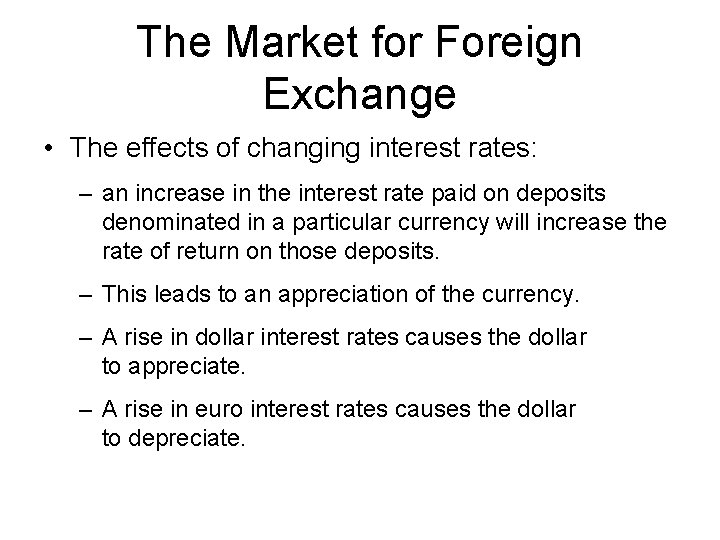The Market for Foreign Exchange • The effects of changing interest rates: – an increase in the interest rate paid on deposits denominated in a particular currency will increase the rate of return on those deposits. – This leads to an appreciation of the currency. – A rise in dollar interest rates causes the dollar to appreciate. – A rise in euro interest rates causes the dollar to depreciate.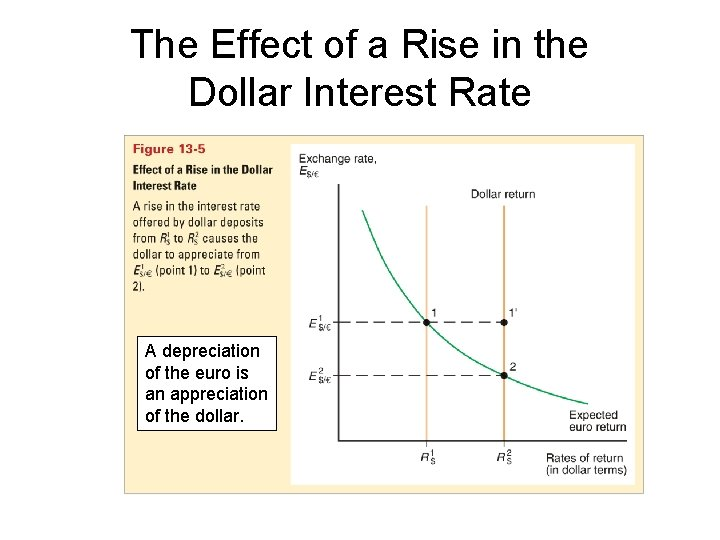The Effect of a Rise in the Dollar Interest Rate A depreciation of the euro is an appreciation of the dollar.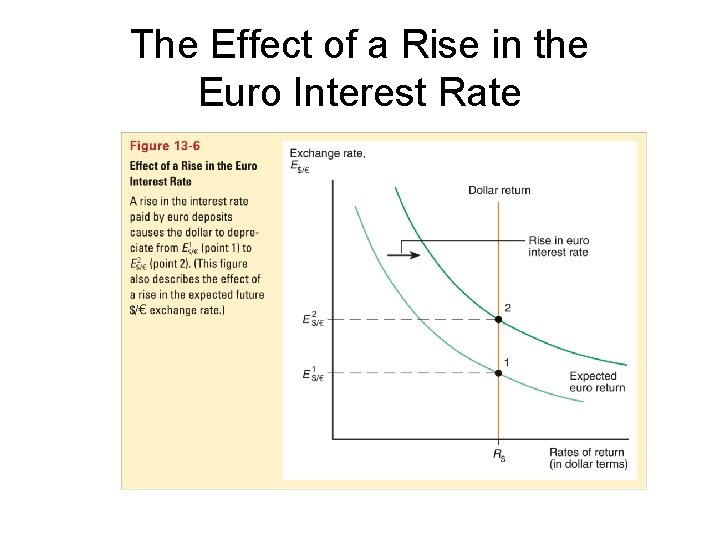The Effect of a Rise in the Euro Interest Rate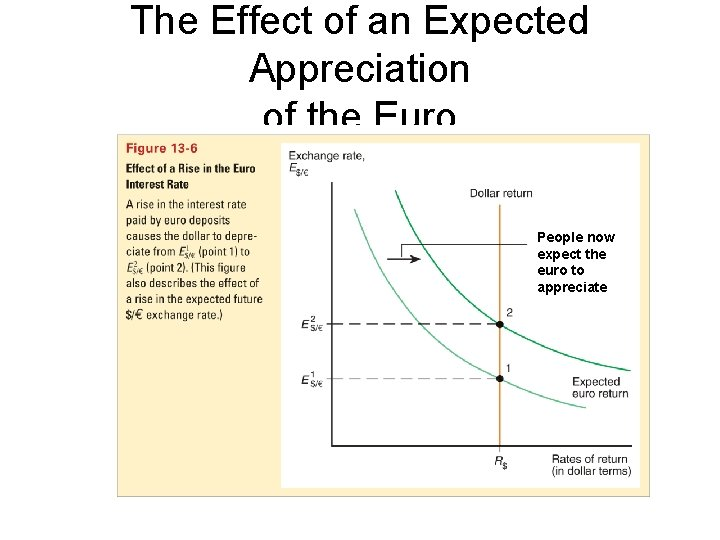The Effect of an Expected Appreciation of the Euro People now expect the euro to appreciateThe Effect of an Expected Appreciation of the Euro (cont. ) • If people expect the euro to appreciate in the future, then investment will pay off in a valuable (“strong”) euro, so that these future euros will be able to buy many dollars and many dollar denominated goods. – the expected return on euros therefore increases. – an expected appreciation of a currency leads to an actual appreciation (a self-fulfilling prophecy) – an expected depreciation of a currency leads to an actual depreciation (a self-fulfilling prophecy)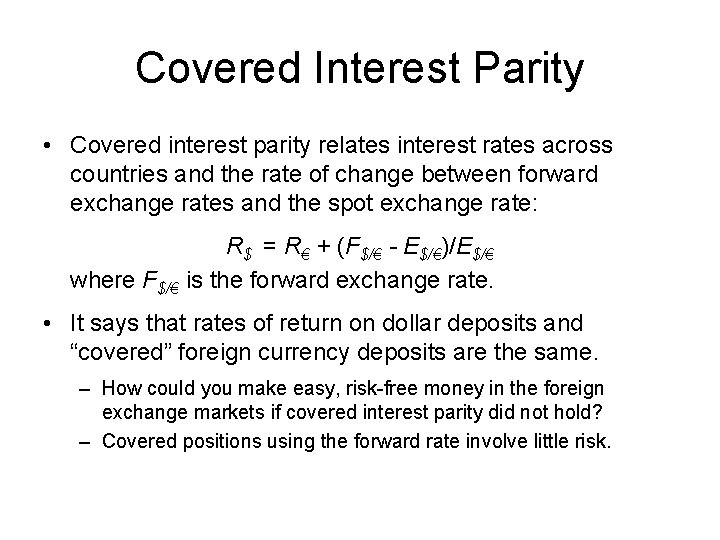Covered Interest Parity • Covered interest parity relates interest rates across countries and the rate of change between forward exchange rates and the spot exchange rate: R\$ = R€ + (F\$/€ - E\$/€)/E\$/€ where F\$/€ is the forward exchange rate. • It says that rates of return on dollar deposits and “covered” foreign currency deposits are the same. – How could you make easy, risk-free money in the foreign exchange markets if covered interest parity did not hold? – Covered positions using the forward rate involve little risk.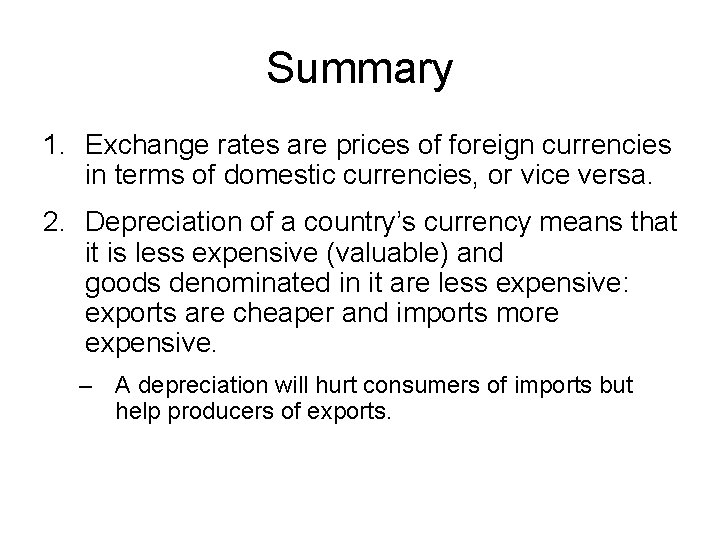Summary 1. Exchange rates are prices of foreign currencies in terms of domestic currencies, or vice versa. 2. Depreciation of a country’s currency means that it is less expensive (valuable) and goods denominated in it are less expensive: exports are cheaper and imports more expensive. – A depreciation will hurt consumers of imports but help producers of exports.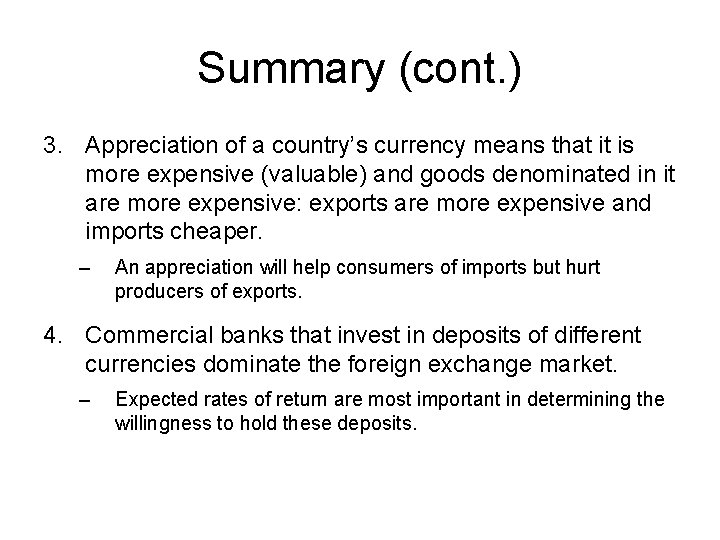Summary (cont. ) 3. Appreciation of a country’s currency means that it is more expensive (valuable) and goods denominated in it are more expensive: exports are more expensive and imports cheaper. – An appreciation will help consumers of imports but hurt producers of exports. 4. Commercial banks that invest in deposits of different currencies dominate the foreign exchange market. – Expected rates of return are most important in determining the willingness to hold these deposits.Summary (cont. ) 5. Returns on bank deposits in the foreign exchange market are influenced by interest rates and expected exchange rates. 6. Equilibrium in the foreign exchange market occurs when returns on deposits in domestic currency and in foreign currency are equal: interest rate parity. 7. An increase in the interest rate on a currency’s deposit leads to an increase in the rate of return and to an appreciation of the currency.Summary (cont. ) 8. An expected appreciation of a currency leads to an increase in the expected rate of return for that currency, and leads to an actual appreciation. 9. Covered interest parity says that rates of return on domestic currency deposits and “covered” foreign currency deposits using the forward exchange rate are the same.